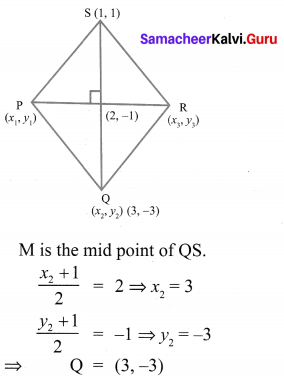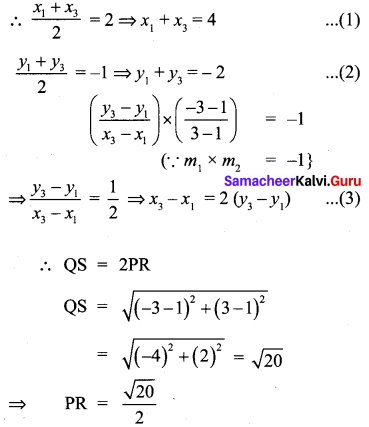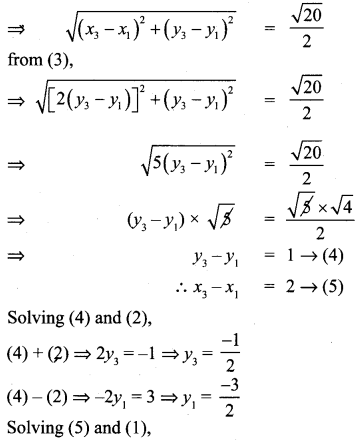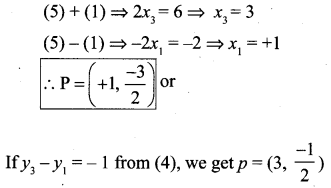## Tamilnadu Samacheer Kalvi 10th Maths Solutions Chapter 5 Coordinate Geometry Ex 5.2

10th Maths Exercise 5.2 Samacheer Kalvi Question 1.
What is the slope of a line whose inclination with positive direction of x -axis is
(i) 90°
(ii) 0°
Solution:
(i) θ = 90°
m = tan θ = tan 90° = ∝ (undefined)
(ii) m = tan θ = tan 0° = 0

Exercise 5.2 Class 10 Samacheer Kalvi Question 2.
What is the inclination of a line whose slope is (i) 0
Solution:
(i) Slope = 0
tan θ = 0
tan 0 = 0
∴ θ = 0°

(ii) Slope = 1
tan θ = 1
tan 45° = 1
∴ θ = 45°
angle of inclination is 45°

Ex 5.2 Class 10 Samacheer Question 3.
Find the slope of a line joining the points
(i) (5, $$\sqrt{5})$$) with origin
(ii) (sin θ, -cos θ) and (-sin θ, cos θ)
(i) (5, $$\sqrt{5})$$) with origin (0, 0)
Solution: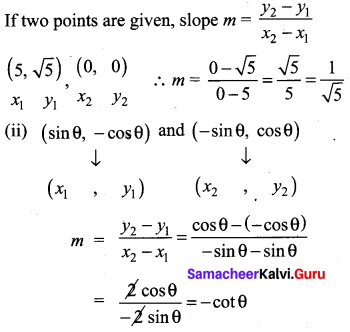10th Maths Exercise 5.2 Question 4.
What is the slope of a line perpendicular to the line joining A(5, 1) and P where P is the mid-point of the segment joining (4, 2) and (-6,4).
Solution:
P is the mid point of the segment joining (4, 2) and (-6, 4)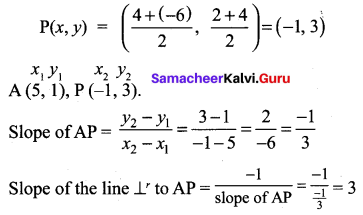10th Maths Ex 5.2 Question 5.
Show that the given points are collinear (-3, -4), (7, 2) and (12, 5)
Solution:
The verticles are A(-3, -4), B(7, 2) and C (12, 5)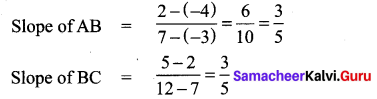Slope of AB = Slope of BC
∴ The points A, B and C lie on the same line.
∴ They are collinear.

10th Maths Exercise 5.2 Solution Question 6.
If the three points (3, -1), (a, 3), (1, -3) are collinear, find the value of a.
Solution:
Slope of AB = slope of BC.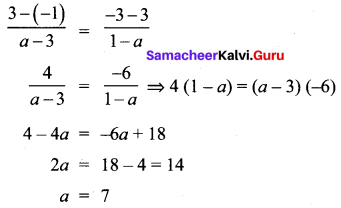Samacheer 10th Maths Exercise 5.2 Solutions Question 7.
The line through the points (-2, a) and (9, 3) has slope –$$\frac{1}{2}$$. Find the value of a.
Solution:
A line joining the points (-2, a) and (9, 3) has slope m = –$$\frac{1}{2}$$.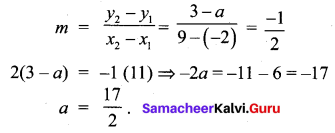10th Maths Coordinate Geometry Exercise 5.2 Question 8.
The line through the points (-2, 6) and (4, 8) is perpendicular to the line through the points (8, 12) and (x, 24) . Find the value of x.
Solution: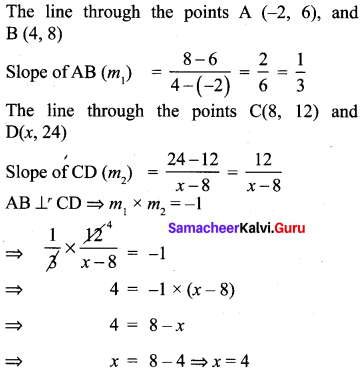10th Maths Exercise 5.2 In Tamil Question 9.
Show that the given points form a right angled triangle and check whether they satisfies pythagoras theorem
(i) A(1, -4), B(2, -3) and C(4, -7)
(ii) L(0, 5), M(9, 12) and N(3, 14)
Solution: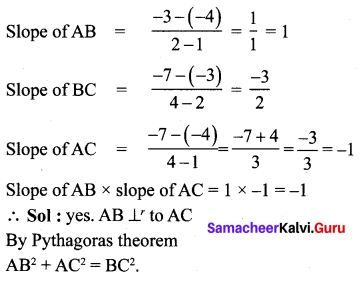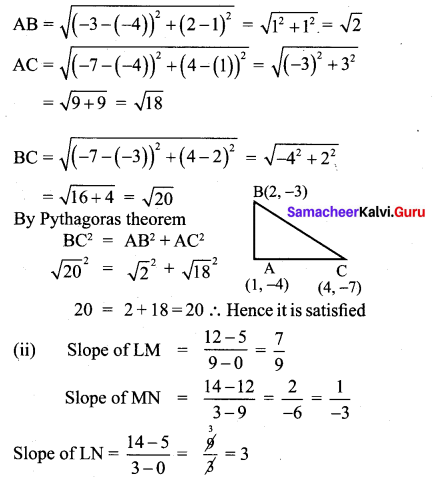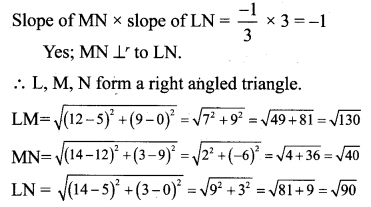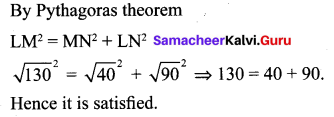10th Coordinate Geometry 5.2 Question 10.
Show that the given points form a parallelogram : A(2.5, 3.5), B(10, -4), C(2.5, -2.5) and D(-5, 5)
Solution: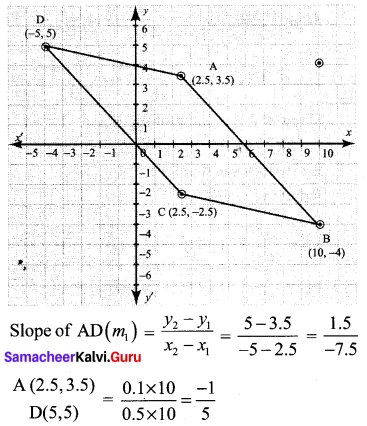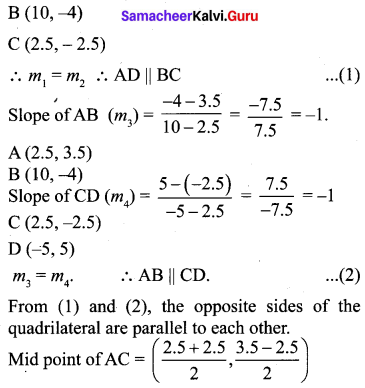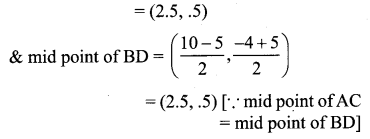∴ The given points form a parallelogram.

10th Maths 5.2 Exercise Question 11.
If the points A(2, 2), B(-2, -3), C(1, -3) and D(x, y) form a parallelogram then find the value of x and y.
Solution:
A(2, 2), B(-2, -3), C(1, -3), D(x, y)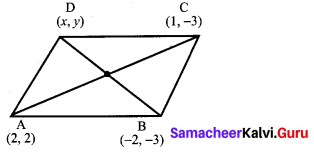Since ABCD forms a parallelogram, slope of opposite sides are equal and diagonals bisect each other.
Mid point of BD = Mid point of AC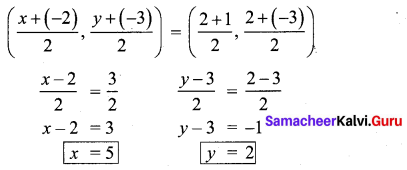10th Maths 5.2 Question 12.
Let A(3, -4), B(9, -4), C(5, -7) and D(7, -7). Show that ABCD is a trapezium.
Solution:
A (3, -4), B (9, -4), C (5, -7) and D (7, -7)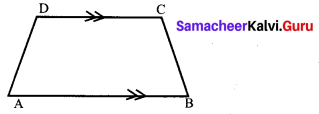If only one pair of opposite sides of a quadrilateral are parallel, then it is said to be a trapezium.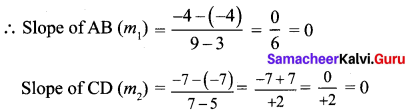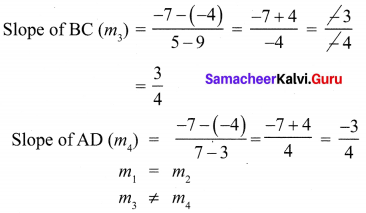∴ One pair of opposite sides are parallel.
∴ ABCD is a trapezium.

Samacheer Kalvi 10th Maths Exercise 5.2 Question 13.
A quadrilateral has vertices at A(- 4, -2), B(5, -1) , C(6, 5) and D(-7, 6). Show that the mid-points of its sides form a parallelogram
Solution: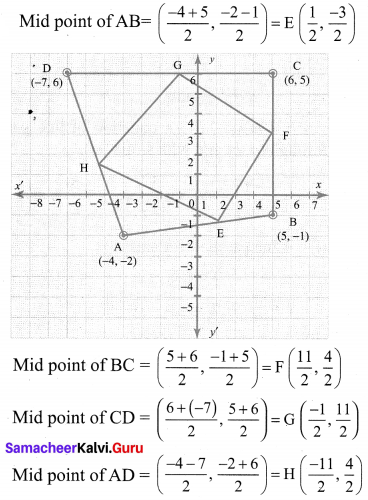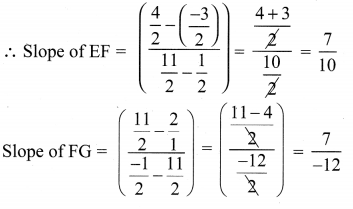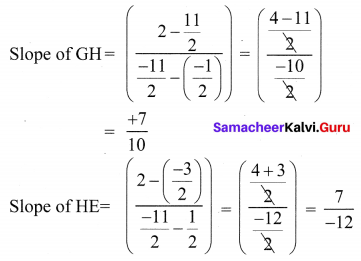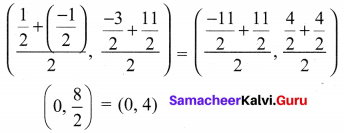In a parallelogram diagonals bisect each other. Opposite sides are parallel as their slopes are equal the mid points of the diagonals are the same.
∴ Mid points of the sides of a quadrilateral form a parallelogram.

10th Exercise 5.2 Question 14.
PQRS is a rhombus. Its diagonals PR and QS intersect at the point M and satisfy QS =2PR. If the coordinates of S and M are (1, 1) and (2, -1) respectively, find the coordinates of P.
Solution: